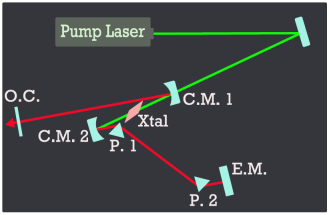/ Denis Seletskiy | Main / ICDC browse

ICDC (Intra-Cavity Dispersion Calculator)λ0 (μm): Δλ (μm): Prism type: FQ1 SF10 Lc (mm): Dp (cm): 4 Lp (mm):Overview:

This program calulates group velocity dispersion (GVD) in a laser cavity containing lasing medium (titanium doped sapphire crystal) and dispersion compensation optics (intra-cavity prism pair). Using this program, user is able to compensate positive dispersion in the lasing crystal by optimizing prism-pair parameters like material, separation and amount of prism-glass inserted into the beam.

Calculation of cavity angle α for astigmatism compensation:

 Crystal length (mm): Mirror curvature (cm): :

Notes:

i) prism insertion calculation is WRONG - stay tuned for updates;

ii) third-order dispersion calculation is absent,

iii) propagation through prisms is assumed to be at minimum deviation angle

User inputs explained:

 λ0 (μm) Center wavelength (in microns) about which the calculation of GVD is centered Δλ (μm) Range of wavelengths for computing GVD (microns) Prism type: FQ1=UV Fused Silica; SF10=Schott SF10 glass Lc (mm) Length of laser crystal in millimeters Dp (cm) Distance between two prisms in centimeters Lp (mm) Total pathlength pulse propagates through prism material in one roundtrip (4 passes).

This application is written in Javascript/DOM with use of Google Chart API to generate plots.

 total number of pageloads: